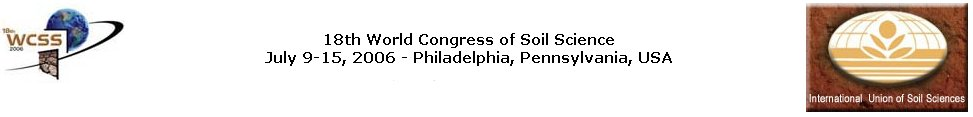### Spatial Modeling of Trace Elements in Soils Using Partial Least Squares Regression and Pre-existing Information.

Hocine Bourennane, National Institut for Agronomic Research (INRA), Avenue de la pomme de pin, BP 20619 Ardon, Orleans, 45166, France

Mapping the pedogeochemical background requires numerous and expensive Trace Element (TE) measurements. One approach consists in using relationships between TE and other soil variables to improve spatial prediction of target variables. Soil Variables (SV) such as granulometric fractions, organic carbon content, CEC, major elements concentration (Fe, Mn, K, Ca…) are much more easy to determine and cheaper to analyze. Moreover, these variables are a direct expression of the pedological processes and thus, may represent the TE behavior. A complementary approach consists on using pre-existing soil maps in order to take into account pedological processes underlying spatial variability of TE. The aim of the study is thus to assess the possibility of inferring trace element content of soils using major physico-chemical soil variables and soil unit delineations. These two auxiliary data include knowledge on underlying pedogenetic processes. As many soil properties, TE and SV are severely corrupted by noise and/or colinearities among a high number of variables. To go beyond these problems, it is convenient to apply latent variable models, particularly Partial Least Squares (PLS) regression modeling. PLS maximizes the covariance between process variables and response variables. In addition, PLS uses only the variation in the X matrix (process variables: SV in our study) that is significant in predicting the variation in the Y matrix (response variables: TE in our study). PLS regression searches for a set of components (called latent vectors: LVs) that performs a simultaneous decomposition of X and Y with the constraint that these components explain as much as possible of the covariance between X and Y. The study area is (“La Châtre” 1:50,000 sheet) located in center of France (North of Massif Central) in a context of marine sedimentary and metamorphic rocks. In a first step, the behavior and the relationships among variables were summarized through kriged maps of the first two LVs of the PLS, which capture most of the systematic variation. The method was applied to punctual data sets giving a first attempt of the TE spatial variability. In a second step, a spatial constraint, according to the soil mapping units (soil series), was introduced in the kriging equations. To reduce the number of soil mapping units, the latter were merged in wider pedo-geological families (PGF). Thus, a second attempt of the TE spatial variability was obtained taking into account both the pedogenetic knowledge included in the choice of basic data (i.e. process variables), and the synthetic representation of the spatial soil variability (i.e. soil map). Due to a limited number of TE measurements, validation of both models was firstly performed by cross-validation. Secondly, mapping results were compared to geochemical background maps achieved by stream sediment measurements at the outlet of catchments. This comparison between average soil TE content per catchment, and corresponding stream TE content showed the consistency between the two independent data sources. It allows finally discussion on catchment behaviors according to the complexity of soil, and landscape parameters over the study area.